Important Formulas & Examples: Trigonometric Functions - 1

# Important Formulas & Examples: Trigonometric Functions - 1 - Mathematics (Maths) Class 11 - Commerce

 Table of contentsTrigonometric RatiosBasic Trigonometric IdentitiesTrigonometric Functions of Allied AnglesTrigonometric Functions of Sum Or Difference of Two AnglesMultiple Angles & Sub-Multiple AnglesConditional IdentitiesMaximum & Minimum values of Trigonometric FunctionsIn mathematics, trigonometry is one of the most important topics to learn. Trigonometry is the study of triangles, where "Trigon" means triangle and "metry" means measurement. Below is a list of formulas based on the right triangle that can be used for studying trigonometry.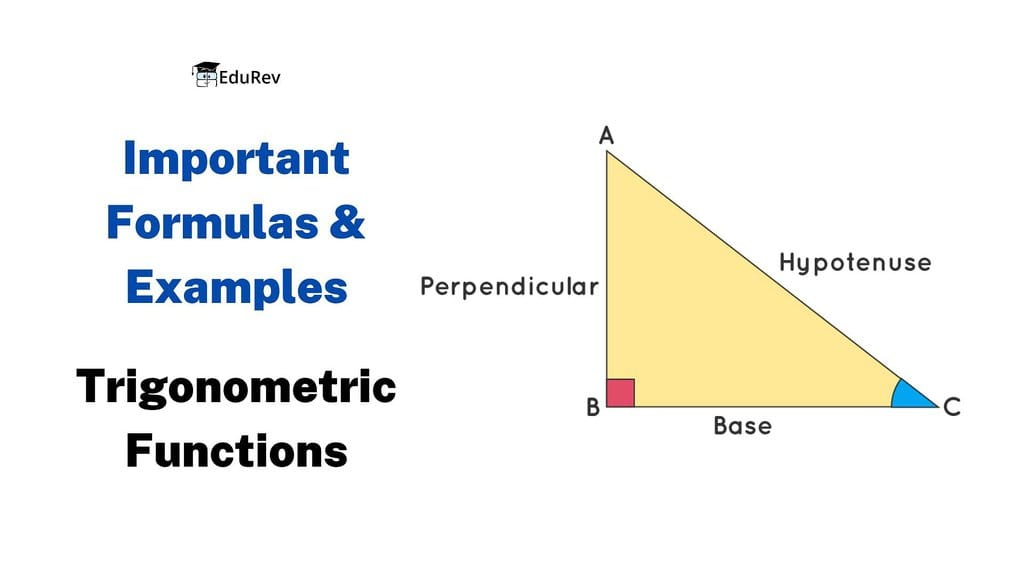## Trigonometric Ratios

The three main functions in trigonometry are Sine, Cosine and Tangent. They are just the length of one side divided by another

Consider a right-angled triangle with an angle θ, a hypotenuse, a side adjacent to angle θ, and a side opposite the angle to angle θ. For a given angle θ each ratio stays the same no matter how big or small the triangle is

• Sine Function: sin(θ) = Opposite Side / Hypotenuse
• Cosine Function: cos(θ) = Adjacent Side / Hypotenuse
• Tangent Function: tan(θ) = Opposite Side / Adjacent Side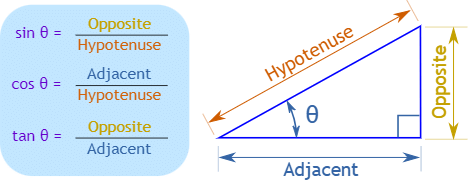When we divide Sine by Cosine we get: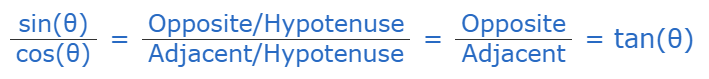• Cosecant Function: cosec(θ) = Hypotenuse / Opposite
• Secant Function: sec(θ) = Hypotenuse / Adjacent

• Cotangent Function: cot(θ) = Adjacent / Opposite

### Trigonometric Ratios Table

The trigonometric table is simply a collection of the values of trigonometric ratios for various angles including 0°, 30°, 45°, 60°, 90°, sometimes with other angles like 180°, 270°, and 360° included, in a tabular format.
Here is the trigonometry table for standard angles along with some non-standard angles: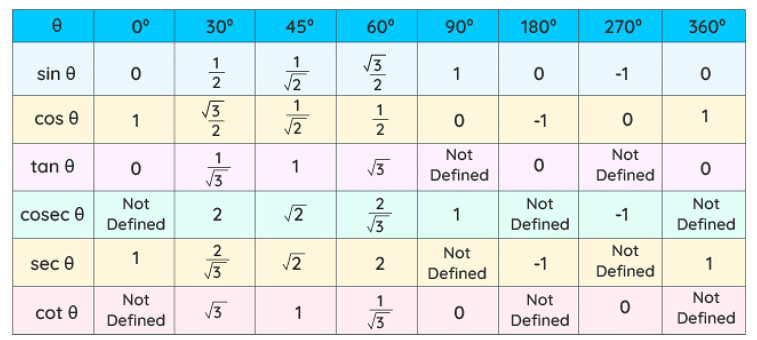## Basic Trigonometric Identities

Tangent and Cotangent Identities

• tan(θ) = sin(θ) / cos(θ)
• cot(θ) = cos(θ) / sin(θ)

Reciprocal Identities

• sin(θ)= 1/cosec(θ)
• cosec(θ) = 1/sin(θ)
• cos(θ) = 1/sec(θ)
• sec(θ) = 1/cos(θ)
• tan(θ) = 1/cot(θ)
• cot(θ) = 1/tan(θ)

Pythagorean Identities

• sin2(θ) + cos2(θ) = 1 ; (-1 ≤ sin θ ≤ 1 ; -1 ≤ cos θ ≤ 1 , θ ∈ R)
• 1 + tan2(θ) = sec2(θ) ; ( |sec θ| ≥ 1, θ ∈ R)
• 1 + cot2(θ) = cosec2(θ)  ; (|cosec θ| ≥ 1 , θ ∈ R)

Even and Odd Angle Formulas

• sin(-θ) = -sin(θ)
• cos(-θ) = cos(θ)
• tan(-θ) = -tan(θ)
• cot(-θ) = -cot(θ)
• sec(-θ) = sec(θ)
• cosec(-θ) = -cosec(θ)

Co-function Formulas

• sin(90°-θ) = cos(θ)
• cos(90°-θ) = sin(θ)
• tan(90°-θ) = cot(θ)
• cot(90°-θ) = tan(θ)
• sec(90°-θ) = cosec(θ)
• cosec(90°-θ) = sec(θ)

Important Trigonometric Ratios

• sin nπ = 0 ; cos nπ = (-1)n ; tan nπ = 0 where n ∈ I
•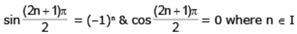•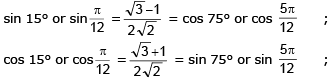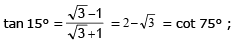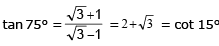•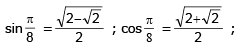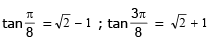•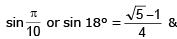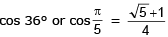## Trigonometric Functions of Allied Angles

If θ is any angle , then - θ, 90 ±θ , 180 ± θ, 270 ± θ , 360 ± θ etc. are called ALLIED ANGLES.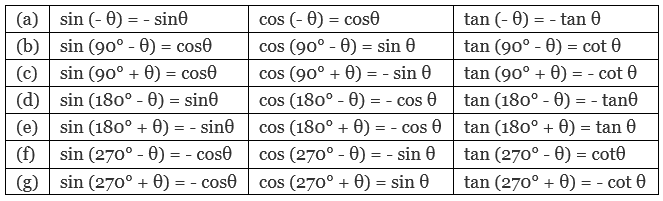Example 1. Express 1·2 radians in degree measure.

1·2 radians =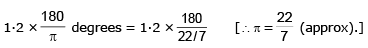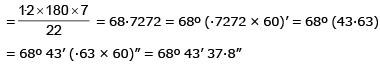Example 2. Calculate sin α if cos α = - 9/11 and α ∈ (π, 3π/2).

For any angle a belonging to the indicated interval sin a is negative, and therefore,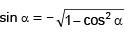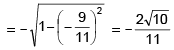Example 3. Calculate tan α if cos α = √5/5 and α ∈ (π, 3π/2)

For any angle a belonging to the indicated interval tanα is positive and cos a is negative, and therefore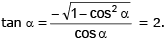## Trigonometric Functions of Sum Or Difference of Two Angles

• sin (A ± B) = sinA cosB ± cosA sinB
• cos (A ± B) = cosA cosB m sinA sinB
• sin²A - sin²B = cos²B - cos²A = sin (A+B) . sin (A- B)
• cos²A - sin²B = cos²B - sin²A = cos (A+B) . cos (A - B)
•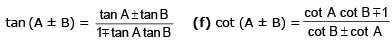Factorisation of the Sum Or Difference of Two sines Or cosines:

• sinC + sinD = 2 sin (C + D/2) cos (C - D/2)
• sinC - sinD = 2 cos (C + D/2) sin (C - D/2)
• cosC + cosD = 2 cos (C + D/2) cos (C - D/2)
• cosC - cosD = - 2 sin (C + D/2) sin (C - D/2)

Transformation of Products Into Sum Or Difference Of sines & cosines:

• 2sinAcosB = sin(A+B) + sin(A-B)
• 2cosAsinB = sin(A+B) - sin(A-B)
• 2cosAcosB = cos(A+B) + cos(A-B)
• 2sinAsinB = cos(A-B) - cos(A+B)

Example 4. Suppose x and y are real numbers such that tan x + tan y = 42 and cot x + cot y = 49. Find the value of tan(x + y).

tan x + tan y = 42 and cot x + cot y = 49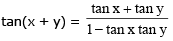now, cot x + cot y = 49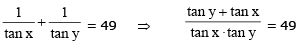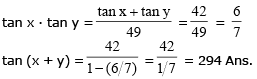Example 5. If x sinθ = y sin(θ + 2π/3) = z sin (θ + 4π/3) then:
(a) x + y + z = 0
(b) xy + yz + zx = 0
(c) xyz + x + y + z = 1
(d) none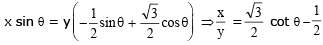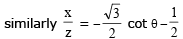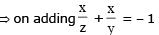= xy + yz + zx = 0

Example 6. Find θ satisfying the equation, tan 15° . tan 25° . tan 35° = tan 0, where θ ∈ (0, 15°).

LHS = tan 15° . tan (30° - 5°) . tan (30° + 5°)
let t = tan 30° and m = tan 5°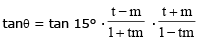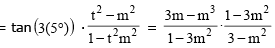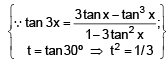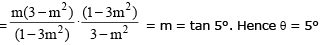Example 7. If tan A & tan B are the roots of the quadratic equation, a x2 + b x + c = 0 then evaluate a sin2 (A + B) + b sin (A + B) . cos (A + B) + c cos2 (A + B).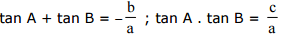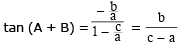Now E = cos2 (A + B) [a tan2 (A + B) + b tan (A + B) + c]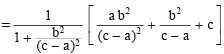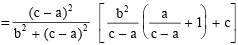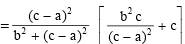Example 8. Show that cos2A + cos2(A + B) + 2 cosA cos(180° + B) · cos(360° + A + B) is independent of A. Hence find its value when B = 810°.

cos2A + cos2(A + B) - [2 cosA · cosB · cos (A + B)]
= cos2A + cos2(A + B) - [ {cos(A + B) + cos(A - B) } cos (A + B) ]
= cos2A + cos2(A + B) - cos2(A + B) - (cos2A - sin2B)
= sin2B which is independent of A
now,  sin2(810°) = sin2(720° + 90°) = sin290° = 1

## Multiple Angles & Sub-Multiple Angles

• sin 2A = 2 sinA cosA ; sin θ = 2 Sin(θ/2)Cos(θ/2)
• cos 2A = cos²A - sin²A = 2cos²A - 1 = 1 - 2 sin²A ;
cos θ= cos² θ/2 - sin² θ/2 = 2cos² θ/2 - 1 = 1 - 2sin² θ/2.
2 cos²A = 1 + cos 2A , 2sin²A = 1 - cos 2A
2 cos² θ/2 = 1 + cos θ, 2 sin² θ/2 = 1 - cos θ.
•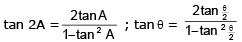•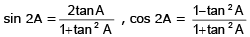• cos 3A = 4 cos3A - 3 cosA
• sin 3A = 3 sinA - 4 sin3A
•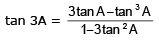## Conditional Identities

If A+B+C = π

• tanA + tanB + tanC = tanA tanB tanC
•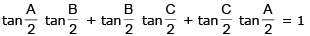• sin2A + sin2B + sin2C = 4 sinA sinB sinC
• sinA + sinB + sinC = 4 cos A/2 Cos B/2 Cos C/2
• cos 2A + cos 2B + cos 2C = –1 – 4 cos A cos B cos C
• cos A + cos B + cos C = 1 + 4 sin A/2 sin B/2 sin C/2

Example 10. If A + B + C = π, prove that,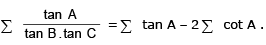Solution: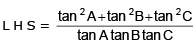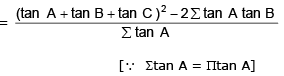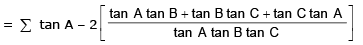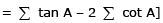Example 11. If A + B + C = θ and cotθ = cot A + cot B + cot C, show that , sin(A-θ). sin (B-θ).sin (C-θ)= sin3 θ.

Solution:

Given cot θ = cot A + cot B + cot C or cot θ - cot A = cot B + cot C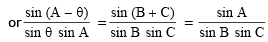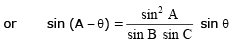....(1)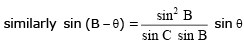....(2)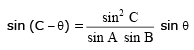....(3)

Multiplying (1) , (2) and (3) we get the result

Example 12. Find whether a triangle ABC can exist with the tangents of its interior angle satisfying, tan A = x, tan B = x + 1, and tan C = 1 - x for some real value of x. Justify your assertion with adequate reasoning.

Solution:

In a triangle ∑ tan A = π tan A (to be proved)
x + x + 1 + 1 – x = x(1 + x)(1 – x)
2 + x = x – x3; x3 = –2, x = -21/3
Hence tanA = x < 0 and tanB = x + 1 = 1 – 21/3 < 0
Hence A and B both are obtuse. Which is not possible in a triangle. Hence no such triangle can exist.

## Maximum & Minimum values of Trigonometric Functions

• Min. value of a2 tan2 θ + b2 cot2θ = 2ab
• Max and Min. value of acosθ + bsinθ are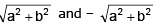• If f(θ) = acos(α + q) + bcos(β + q) where a, b, α and β are known quantities then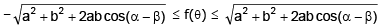•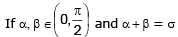(constant) then the maximum values of the expression cosα cosβ, cosα+ cosβ, sinα + sinβ and sina sinb occurs when α = β = σ/2
• If A, B, C are the anlges of a triangle then maximum value of sinA + sinB + sinC and sinA sinB sinC occurs when A = B = C = 60º
• In case a quadratic in sinq or cosq is given then the maximum or minimum values can be interpreted by making a perfect square

Example 13. Find the greatest value of c such that system of equations x2 + y2 = 25; x + y = c has a real solution.

Solution:

Put x = 5 cosθ and y = 5 sinθ

5(cosθ+ sinθ) = c; but (cosθ + sinθ)max = √2 and (cosθ + sinθ)min = – √2

hence, cmax = 5√2

Example 14. Find the minimum and maximum value of f (x, y) = 7x2 + 4xy + 3y2 subjected to x2 + y2 = 1.

Solution:

Let x = cosθ and y = sinθ
y = f (θ) = 7 cos2θ + 4 sin θcosθ + 3 sin2θ = 3 + 2 sin 2q + 2(1 + cos 2θ)
= 5 + 2(sin 2θ + cos 2θ) but -√2 ≤ (sin 2θ + cos 2θ) ≤ √2
ymax = 5 + 2√2 and ymin = 5 – 2√2

The document Important Formulas & Examples: Trigonometric Functions - 1 | Mathematics (Maths) Class 11 - Commerce is a part of the Commerce Course Mathematics (Maths) Class 11.
All you need of Commerce at this link: Commerce

## FAQs on Important Formulas & Examples: Trigonometric Functions - 1 - Mathematics (Maths) Class 11 - Commerce

 1. What are the basic trigonometric identities?Ans. The basic trigonometric identities include: $\sin^2\theta+\cos^2\theta=1$, $\tan\theta=\frac{\sin\theta}{\cos\theta}$, $\cot\theta=\frac{\cos\theta}{\sin\theta}$, and $\sec\theta=\frac{1}{\cos\theta}$ and $\csc\theta=\frac{1}{\sin\theta}$.
 2. What are the important trigonometric ratios?Ans. The important trigonometric ratios include: sine ratio, cosine ratio, tangent ratio, cotangent ratio, secant ratio, and cosecant ratio.
 3. What are the trigonometric functions of sum or difference of two angles?Ans. The trigonometric functions of sum or difference of two angles include: $\sin(A \pm B)=\sin A\cos B\pm\cos A\sin B$, $\cos(A \pm B)=\cos A\cos B\mp\sin A\sin B$, and $\tan(A \pm B)=\frac{\tan A\pm\tan B}{1\mp\tan A\tan B}$.
 4. What are the maximum and minimum values of trigonometric functions?Ans. The maximum value of sine and cosine functions is 1, and the minimum value is -1. The maximum value of tangent and cotangent functions is undefined, and the minimum value is -∞ to +∞. The maximum value of secant and cosecant functions is -1 and 1, respectively, and the minimum value is -∞ to +∞.
 5. What are the conditional identities in trigonometry?Ans. The conditional identities in trigonometry include: $\sin(-\theta)=-\sin\theta$, $\cos(-\theta)=\cos\theta$, $\tan(-\theta)=-\tan\theta$, $\sin(\pi-\theta)=\sin\theta$, $\cos(\pi-\theta)=-\cos\theta$, and $\tan(\pi-\theta)=-\tan\theta$.

## Mathematics (Maths) Class 11

157 videos|210 docs|132 tests

## Mathematics (Maths) Class 11

157 videos|210 docs|132 tests
Signup to see your scores go up within 7 days! Learn & Practice with 1000+ FREE Notes, Videos & Tests.
10M+ students study on EduRev
Track your progress, build streaks, highlight & save important lessons and more!(Scan QR code)
Related Searches

,

,

,

,

,

,

,

,

,

,

,

,

,

,

,

,

,

,

,

,

,

;